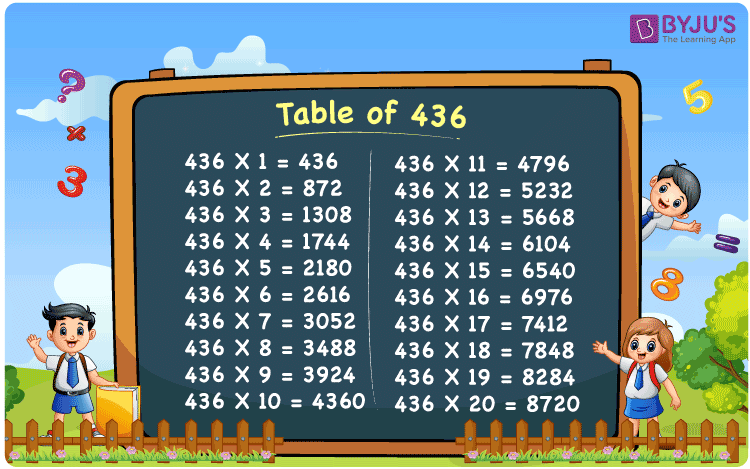Checkout JEE MAINS 2022 Question Paper Analysis : Checkout JEE MAINS 2022 Question Paper Analysis :

# Table of 436

The table of 436 is a multiplication table that is formed by multiplying 436 by natural numbers. Learning the 436 times table is important because it saves time while solving the mathematical problems. Here, we have included a 436 times table chart as well as a tabular version of the multiplication table of 436 on this page. You can download the 436 times table in PDF format by clicking the link below.

## Table of 436 Chart## What is the 436 Times Table?

The repeated addition and multiplication table of 436 is shown below.

 436×1 = 436 436 436×2 = 872 436 + 436 = 872 436×3 = 1308 436 + 436 + 436 = 1308 436×4 = 1744 436 + 436 + 436 + 436 = 1744 436×5 = 2180 436 + 436 + 436 + 436 + 436 = 2180 436×6 = 2616 436 + 436 + 436 + 436 + 436 + 436 = 2616 436×7 = 3052 436 + 436 + 436 + 436 + 436 + 436 + 436 = 3052 436×8 = 3488 436 + 436 + 436 + 436 + 436 + 436 + 436 + 436 = 3488 436×9 = 3924 436 + 436 + 436 + 436 + 436 + 436 + 436 + 436 + 436 = 3924 436×10 = 4360 436 + 436 + 436 + 436 + 436 + 436 + 436 + 436 + 436 + 436 = 4360

## Multiplication Table of 436

The table of 436 is shown below in tabular format.

 436 × 1 = 436 436 × 2 = 872 436 × 3 = 1308 436 × 4 = 1744 436 × 5 = 2180 436 × 6 = 2616 436 × 7 = 3052 436 × 8 = 3488 436 × 9 = 3924 436 × 10 = 4360 436 × 11 = 4796 436 × 12 = 5232 436 × 13 = 5668 436 × 14 = 6104 436 × 15 = 6540 436 × 16 = 6976 436 × 17 = 7412 436 × 18 = 7848 436 × 19 = 8284 436 × 20 = 8720

## Solved Example on the Table of 436

Example:

Calculate the missing value: 436 × ____ = 6976.

Solution:

Let the missing value be “h”.

I.e., 436h = 6976

h = 6976/436

h = 16

Thus, the missing value is 16.

I.e., 436 × 16 = 6976.

## Frequently Asked Questions on the Table of 436

### What is the table of 436?

The table of 436 gives the multiples of 436, which is obtained by multiplying 436 with the natural numbers.

### Write the 436 times table.

436×1 = 436, 436×2 = 872, 436×3 = 1308, 436×4 = 1744, 436×5 = 2180, 436×6 = 2616, 436×7 = 3052, 436×8 = 3488, 436×9 = 3924, 436×10 = 4360.

### What is 436 times 15?

436 times 15 is 6540. I.e., 436 × 15 = 6540.